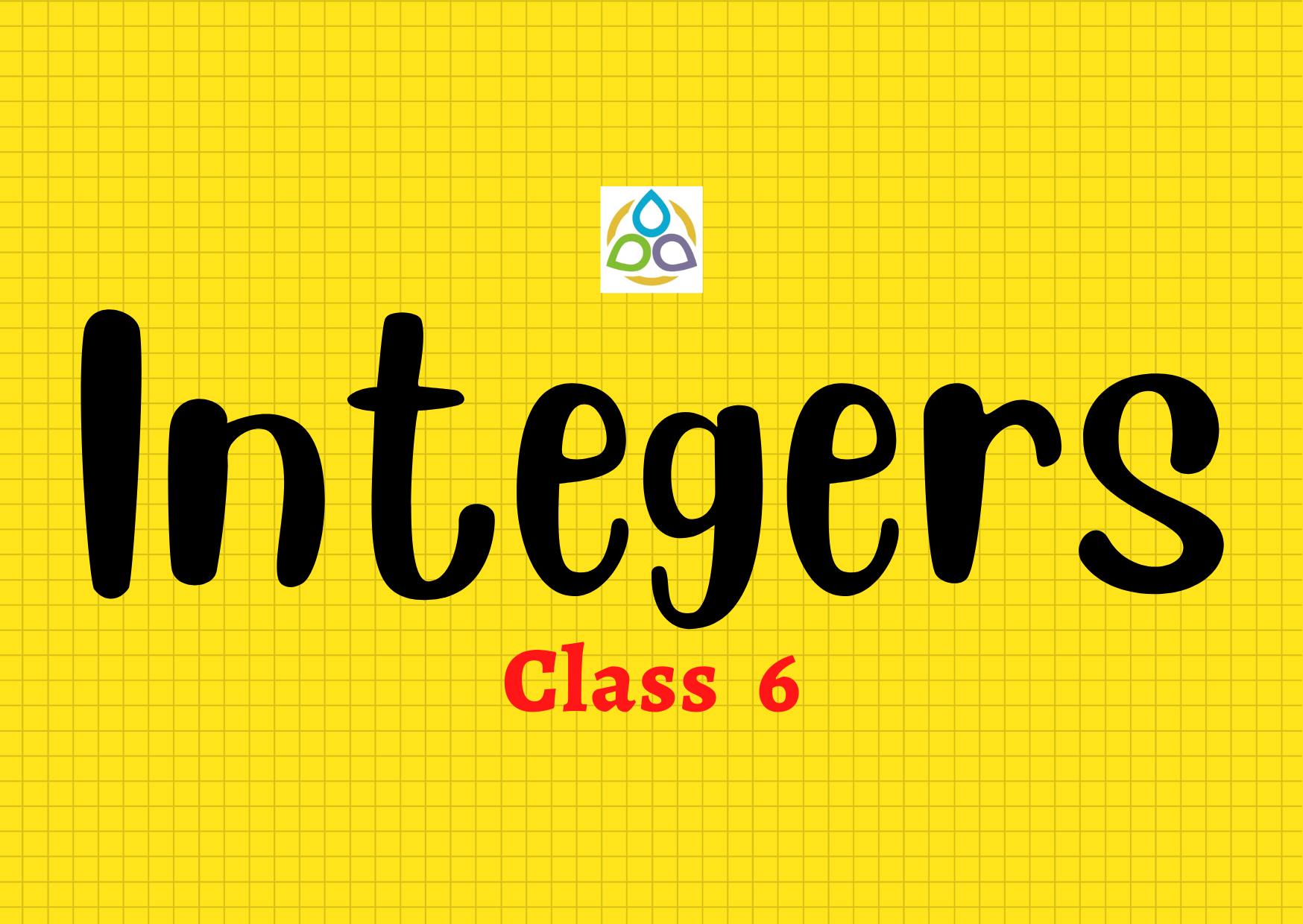# Integers

50. What is the set of negative numbers and whole numbers called?

• (A) The set of natural numbers
• (B) The set of integers
• (C) The set of positive numbers
• (D) The set of negative numbers

49. The numbers +1, +2, +3, +4, . . . are referred to as

• (A) Integers
• (B) Positive integers
• (C) Negative integers
• (D) Whole numbers

48. The numbers –1, –2, –3, –4, . . . are referred to as

• (A) Integers
• (B) Positive integers
• (C) Negative integers
• (D) Whole numbers

47. The numbers . . . , –4, –3, –2, –1, 0, +1, +2, +3, . . . are called

• (A) Integers
• (B) Positive integers
• (C) Negative integers
• (D) Non-negative integers

46. Fill in the blank: All the positive integers lie to the __________ of 0

• (A) Right
• (B) Left
• (C) Up
• (D) Down

45. Fill in the blank: All the negative integers lie to the __________ of 0

• (A) Right
• (B) Left
• (C) Up
• (D) Down

44. The non-negative integers are also called

• (A) Natural numbers
• (B) Positive integers
• (C) Negative integers
• (D) Whole numbers

43. The sum of two negative integers is again a

• (A) Natural number
• (B) Positive integer
• (C) Negative integer
• (D) Whole number

42. The difference of two negative integers is

• (A) A natural number
• (B) A positive integer
• (C) A non-negative integer
• (D) Depend on the numbers

41. The sum of a positive integer and a negative integer is always

• (A) A natural number
• (B) A positive integer
• (C) A negative integer
• (D) An integer

40. When a negative integer is subtracted from another negative integer, the sign of the result

• (A) is always negative
• (B) is always positive
• (C) is never negative
• (D) depends on the numerical value of the integers

39. The statement When an integer is added to itself, the sum is greater than the integer is

• (A) always true
• (B) never true
• (C) true only when the integer is positive
• (D) true for non-negative integers

38. Two integers are said to be additive inverses of each other if

• (A) the sum of them is zero
• (B) the sum of them is 1
• (C) the sum of them is −1
• (D) the product of them is –1

37. The additive inverse of a negative integer

• (A) is always negative
• (B) is always positive
• (C) is the same integer
• (D) zero

36. The additive inverse of 0 is _______.

• (A) 0
• (B) –0
• (C) 1
• (D) –1

35. The additive inverse of –1 is _______.

• (A) 0
• (B) 1
• (C) 10
• (D) –1